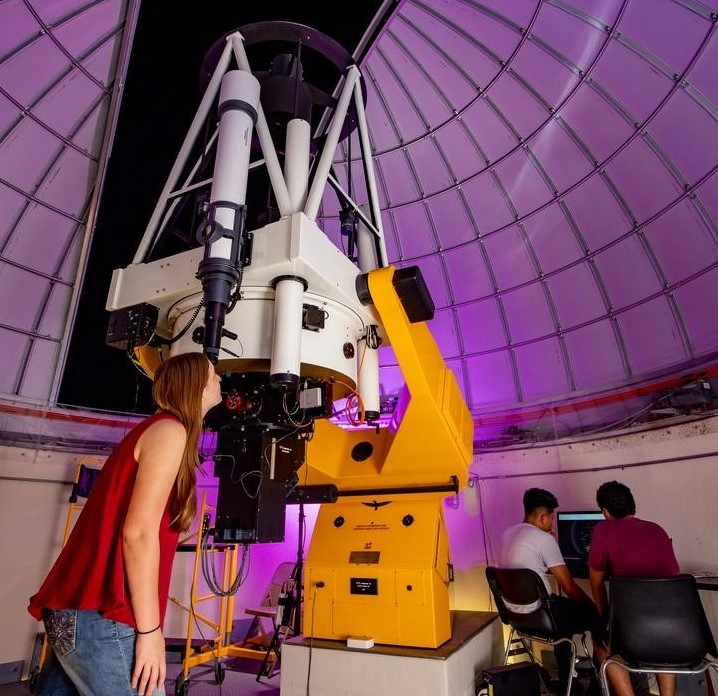## Aerospace, Physics, and Space Science Faculty Publications

### Gaussian reference Auid and interpretation of quantum geometrodynamics

Article

#### Publication Title

Physical Review D.

#### Abstract

The of vacuum geometrodynamics is turned into a Schrödinger equation by imposing the normal Gaussian coordinate conditions with s and then restoring the coordinate invariance of the action by parametrization. This procedure corresponds to coupling the gravitational field to a reference fluid. The source appearing in the Einstein law of gravitation has the structure of a heat-conducting dust. When one imposes only the Gaussian time condition but not the Gaussian frame conditions, the heat flow vanishes and the dust becomes incoherent. The canonical description of the fluid uses the Gaussian coordinates and their conjugate momenta as the fluid variables. The energy density and the momentum density of the fluid turn out to be homogeneous linear functions of such momenta. This feature guarantees that the Dirac constraint quantization of the gravitational field coupled to the Gaussian reference fluid leads to a functional Schrödinger equation in Gaussian time. Such an equation possesses the standard positive-definite conserved norm. For a heat-conducting fluid, the states depend on the metric induced on a given hypersurface; for an incoherent dust, they depend only on geometry. It seems natural to interpret the integrand of the norm integral as the probability density for the metric (or the geometry) to have a definite value on a hypersurface specified by the Gaussian clock. Such an interpretation fails because the reference fluid is realistic only if its energy-momentum tensor satisfies the familiar energy conditions. In the canonical theory, the energy conditions become additional constraints on the induced metric and its conjugate momentum. For a heat-conducting dust, the total system of constraints is not first class and cannot be implemented in quantum theory. As a result, the Gaussian coordinates are not determined by physical properties of a realistic material system and the probability density for the metric loses thereby its operational significance. For an incoherent dust, the energy conditions and the dynamical constraints are first class and can be carried over into quantum theory. However, because the geometry operator considered as a multiplication operator does not commute with the energy conditions, the integrand of the norm integral still does not yield the probability density. The interpretation of the Schrödinger geometrodynamics remains viable, but it requires a rather complicated procedure for identifying the fundamental observables. All our considerations admit generalization to other coordinate conditions and other covariant field theories. © 1991 The American Physical Society.

419

441

#### DOI

10.1103/PhysRevD.43.419

1-15-1991

COinS